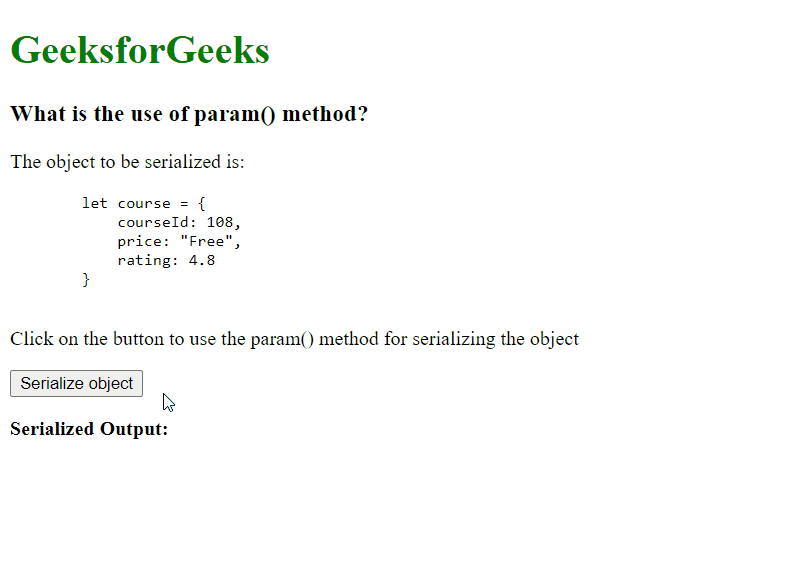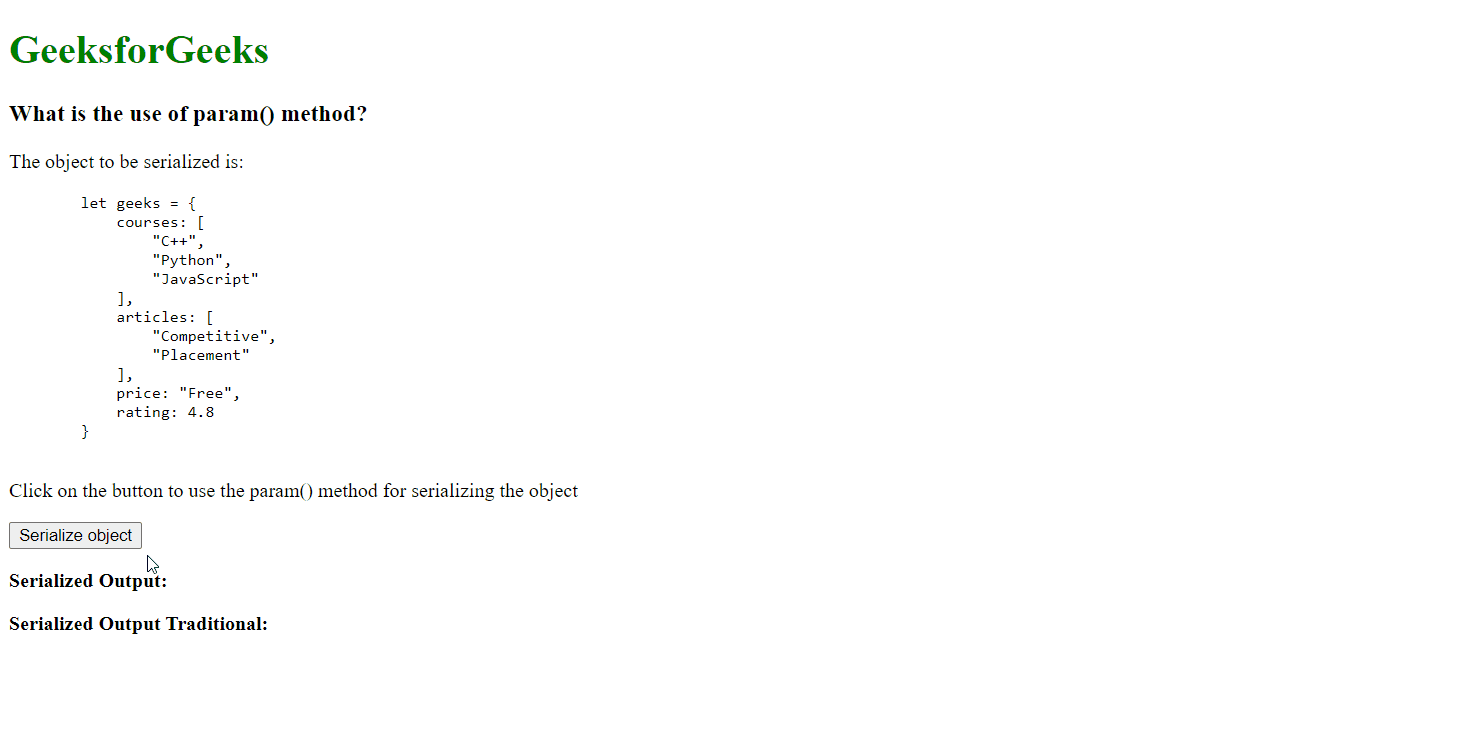# What is the use of param() method in jQuery ?

• Last Updated : 23 Mar, 2022

In this article, we will learn about the param() method which is an inbuilt method provided by jQuery. This method is used for creating a serialized representation of an object. The method will recursively serialize deep objects for trying to work with modern languages like Ruby on Rails or Python.

This is helpful in situations where an object’s data has to be passed to a server in the form of a string, like during an Ajax request. This method also supports the traditional method of serialization if required. The traditional method prevents the use of brackets in the serialized form.

Syntax:

`\$.param( obj, traditional )`

Parameters: This method accepts two parameters as mentioned above and described below:

• obj: This is used to specify an array, object or jQuery object to serialize. It is required that the parameter is properly serializable.
• traditional: This is used to specify if the traditional method of serialization has to be used. It is an optional parameter.

Return Value: It returns a string that contains the serialized form of the given object.

Example 1: This example use param() method to create serialized representation of an object.

## HTML

 ` ` `<``html``> ` ` `  `<``head``> ` `    ``<``script` `src``= ` `"https://code.jquery.com/jquery-3.6.0.min.js"``> ` `    `` ` ` ` ` `  `<``body``> ` `    ``<``h1` `style``=``"color: green"``>GeeksforGeeks ` `    ``<``h3``>What is the use of param() method? ` ` `  `    ``<``p``>The object to be serialized is: ` `    ``<``pre``> ` `        ``let course = { ` `            ``courseId: 108, ` `            ``price: "Free", ` `            ``rating: 4.8 ` `        ``} ` `    `` ` `     `  `    ``<``p``>Click on the button to use the param() ` `       ``method for serializing the object ` ` `  `    ``<``button` `onclick``=``"serializeObject()"``> ` `      ``Serialize object ` `    `` ` ` `  `    ``<``p``><``b``>Serialized Output: ` `    ``<``div` `id``=``"output"``> ` ` `  `    ``<``script` `type``=``"text/javascript"``> ` ` `  `        ``function serializeObject() { ` ` `  `            ``// The object to serialize ` `            ``let course = { ` `                ``courseId: 108, ` `                ``price: "Free", ` `                ``rating: 4.8 ` `            ``} ` ` `  `            ``// Find the serialized output using ` `            ``// the param() method ` `            ``let serializedOutput = \$.param(course); ` `           `  `            ``// Display it on the page ` `            ``\$("#output").text(serializedOutput); ` `        ``} ` `    `` ` ` ` ` `  ``

Output:Example 2: This example use compare the differences when using the traditional parameter.

## HTML

 ` ` `<``html``> ` ` `  `<``head``> ` `    ``<``script` `src``= ` `"https://code.jquery.com/jquery-3.6.0.min.js"``> ` ` ` ` `  `<``body``> ` `    ``<``h1` `style``=``"color: green"``>GeeksforGeeks ` `    ``<``h3``>What is the use of param() method? ` ` `  `    ``<``p``>The object to be serialized is: ` `    ``<``pre``> ` `        ``let geeks = { ` `            ``courses: [ ` `                ``"C++", ` `                ``"Python", ` `                ``"JavaScript" ` `            ``], ` `            ``articles: [ ` `                ``"Competitive", ` `                ``"Placement" ` `            ``], ` `            ``price: "Free", ` `            ``rating: 4.8 ` `        ``} ` `    `` ` ` `  `    ``<``p``>Click on the button to use the param()  ` `       ``method for serializing the object ` ` `  `    ``<``button` `onclick``=``"serializeObject()"``> ` `      ``Serialize object ` `    `` ` ` `  `    ``<``p``><``b``>Serialized Output: ` ` `  `    ``<``div` `id``=``"output"``> ` `    ``<``p``><``b``>Serialized Output Traditional: ` ` `  `    ``<``div` `id``=``"output-traditional"``> ` ` `  `    ``<``script` `type``=``"text/javascript"``> ` `        ``function serializeObject() { ` ` `  `            ``// The object to serialize ` `            ``let geeks = { ` `                ``courses: [ ` `                    ``"C++", ` `                    ``"Python", ` `                    ``"JavaScript" ` `                ``], ` `                ``articles: [ ` `                    ``"Competitive", ` `                    ``"Placement" ` `                ``], ` `                ``price: "Free", ` `                ``rating: 4.8 ` `            ``} ` ` `  `            ``// Find the serialized output using ` `            ``// the param() method and display it ` `            ``\$("#output").text( ` `              ``\$.param(geeks) ` `            ``); ` ` `  `            ``// Using the param() method with the  ` `            ``// traditional parameter set to true ` `            ``// and display it ` `            ``\$("#output-traditional").text( ` `              ``\$.param(geeks, true) ` `            ``); ` `        ``} ` `    `` ` ` ` ` `  ``

Output:Reference: https://api.jquery.com/jquery.param/

My Personal Notes arrow_drop_up
Recommended Articles
Page :